﻿ 一维有源相扫串馈形式阵列雷达测角误差分析
«上一篇文章快速检索 高级检索

 哈尔滨工程大学学报2019, Vol. 40Issue (4): 839-843  DOI: 10.11990/jheu.2017100140

### 引用本文WANG Yanbao, HAO Xiaoning, DONG Guanming, et al. Analysis on error of 1-dimension active phase scanning series-feed array radar angle measurement[J]. Journal of Harbin Engineering University, 2019, 40(4), 839-843. DOI: 10.11990/jheu.201710014.### 文章历史

1. 西安电子工程研究所 目标指示雷达总体部, 陕西 西安 710100;
2. 哈尔滨工程大学 科学技术研究院, 黑龙江 哈尔滨 150001

Analysis on error of 1-dimension active phase scanning series-feed array radar angle measurement
WANG Yanbao 1, HAO Xiaoning 1, DONG Guanming 2, ZHOU Yongwei 1
1. General System Department on Target Designation Radar, Xi'an Electronic Engineering Research Institute, Xi'an 710100, China;
2. Research Institute of Science and Technology, Harbin Engineering University, Harbin 150001, China
Abstract: To solve the problem of radar azimuth and pitching angle errors caused by a wide range of pitching angles, we propose a large dispersion angle and radar tilt angle for a 1D active-phase scanning radar.According to the relationship between the angles, the relational formula between azimuth, pitching angle and dispersion angle, and radar tilt angle of the 1-D active phase scanning can be derived.Compared with conventional correction methods, the proposed method does not need complex coordinate conversion, and the amount of calculation is small, which is convenient for engineering applications.Through analysis of radar flight detection data, the correctness and effectiveness of the correction algorithm are verified, and the angular measurement accuracy of similar radar products can be improved further.
Keywords: 1-dimension active-phase array radar    frequency sweep    string feed    dispersion angle    tilt angle    angle measurement accuracy    angle measurement error    angle correction

1 坐标系定义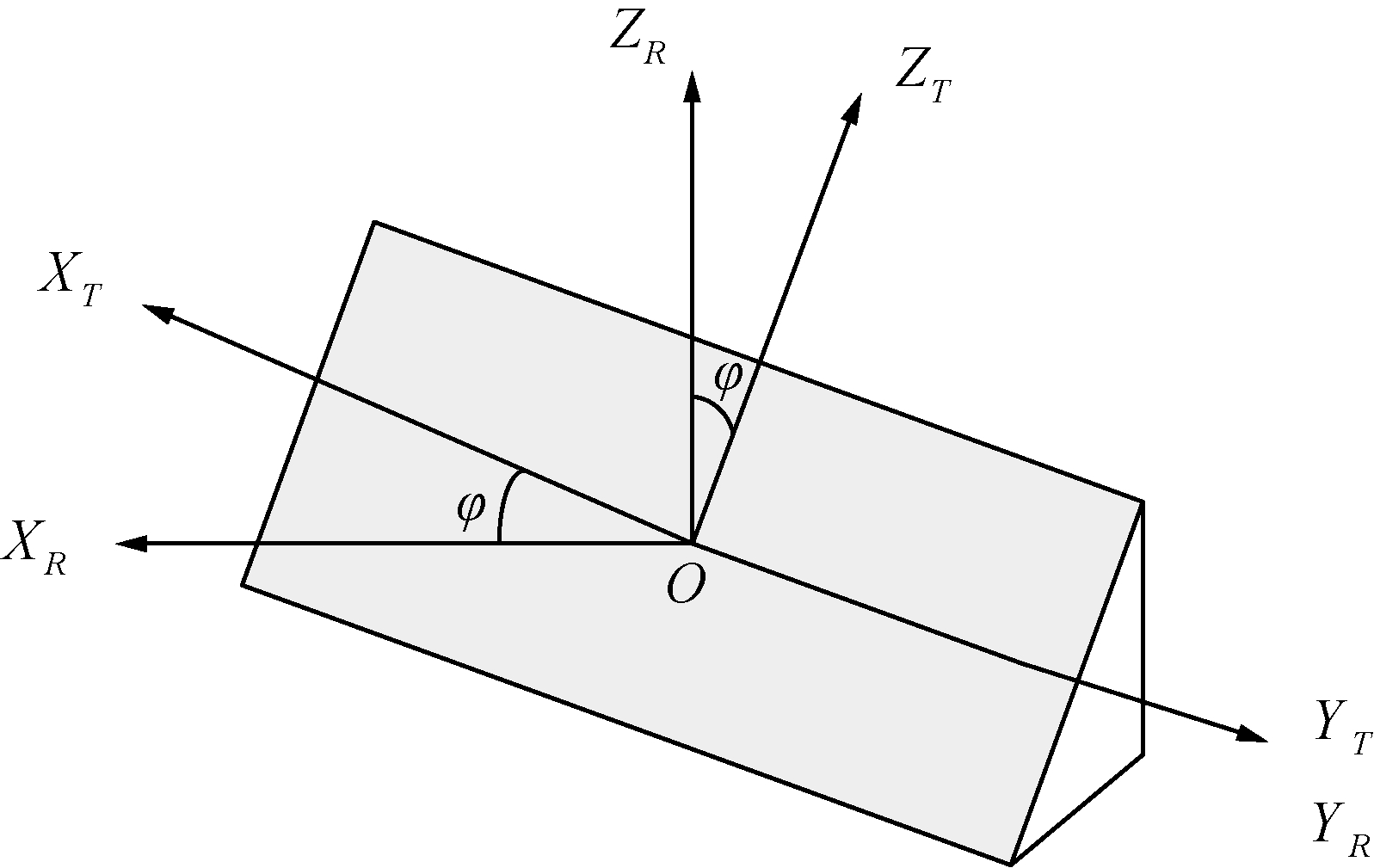Download: 图 1 直角坐标系定义示意 Fig. 1 Rectangular coordinate definition diagram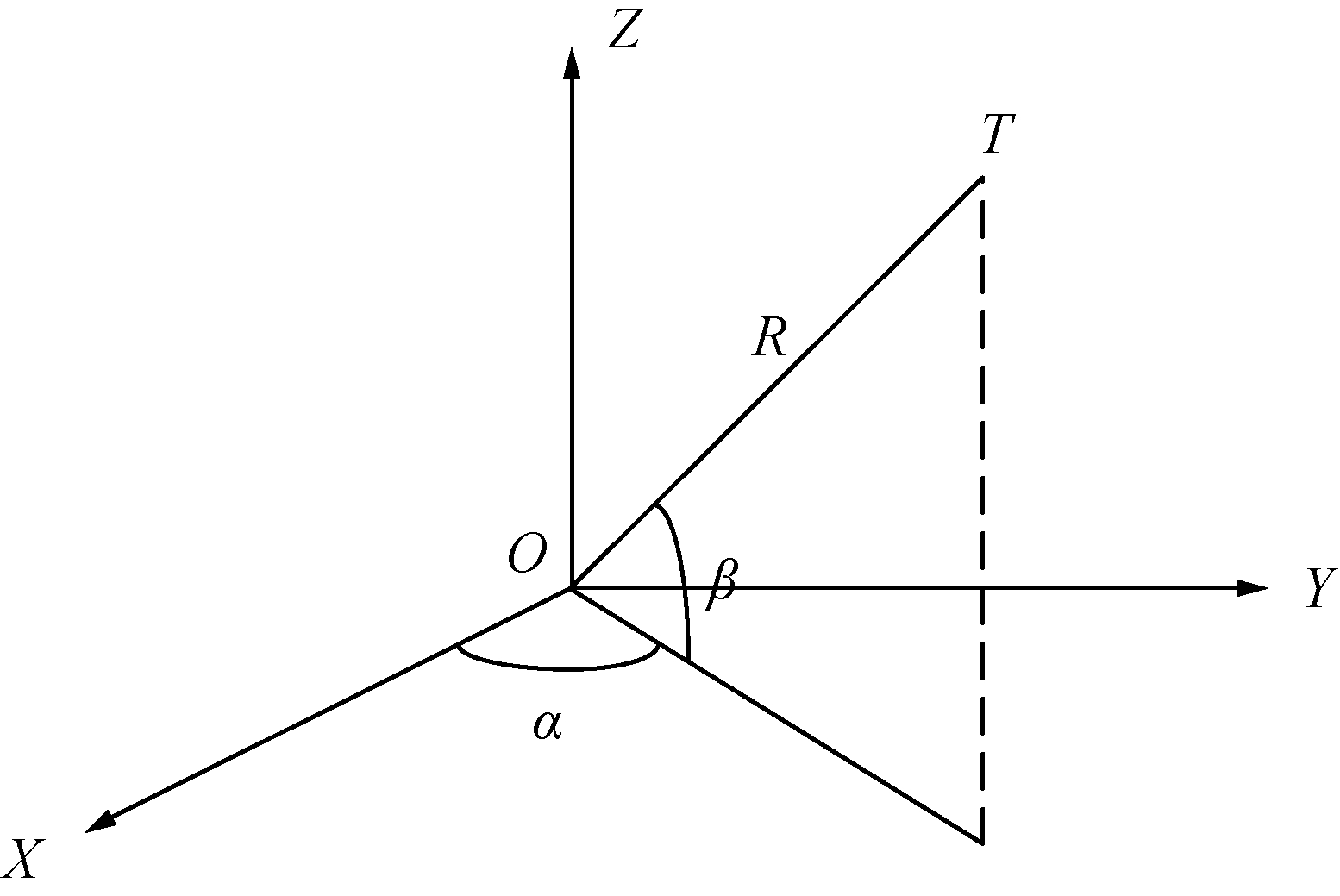Download: 图 2 球坐标系定义示意 Fig. 2 Spherical coordinate definition diagram

2 测角误差源分析与研究 2.1 色散角导致的方位、俯仰测角误差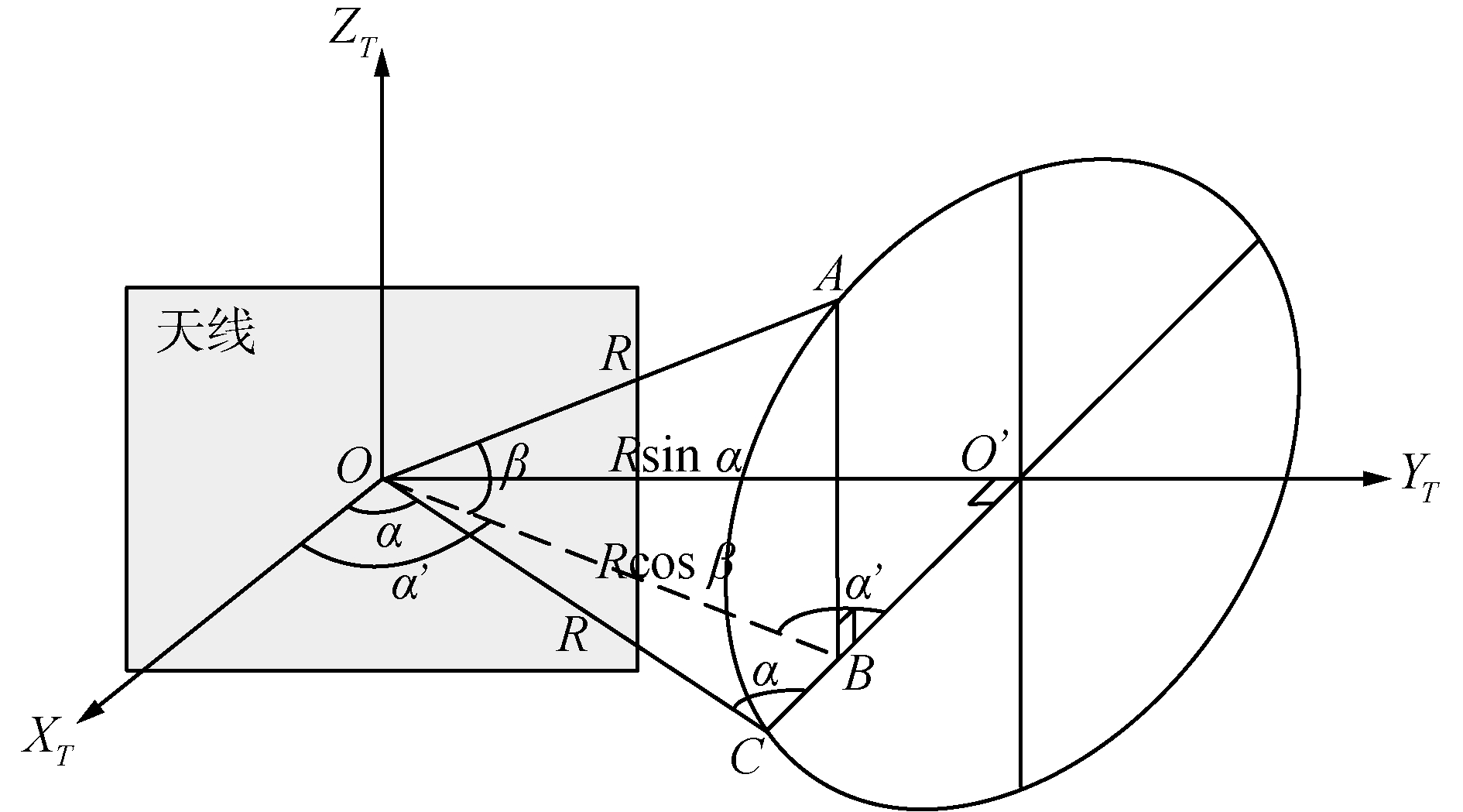Download: 图 3 色散角导致的测角误差几何关系 Fig. 3 Geometric relationship of the angle error due to dispersion angle

 $\alpha ^\prime = {\rm{arcsin}}\left( {\frac{{{\rm{sin}}\alpha }}{{{\rm{cos}}\beta }}} \right)$ (1)

2.2 天线倾斜导致的方位、俯仰测角误差

 $\left\{ \begin{array}{l} {\rm{sin}}\theta = \frac{{AB}}{{AO^\prime }} = \frac{{{\rm{sin}}\beta }}{{{\rm{cos}}\alpha }}\\ \theta = {\rm{arcsin}}\left( {\frac{{{\rm{sin}}\beta }}{{{\rm{cos}}\alpha }}} \right) \end{array} \right.$ (2)
 $\left\{ \begin{array}{l} {\rm{tan}}\alpha ^\prime = \frac{{OO^\prime }}{{O^\prime D}} = \frac{{{\rm{sin}}\alpha }}{{{\rm{cos}}\alpha {\rm{cos}}\left( {\theta + \varphi } \right)}}\\ \alpha ^\prime = {\rm{arctan}}\left( {\frac{{{\rm{sin}}\alpha }}{{{\rm{cos}}\alpha {\rm{cos}}\left( {\theta + \varphi } \right)}}} \right) \end{array} \right.$ (3)
 $\left\{ \begin{array}{l} {\rm{sin}}\beta ^\prime = \frac{{AD}}{{AO}} = {\rm{cos}}\alpha {\rm{sin}}\left( {\theta + \varphi } \right)\\ \beta ^\prime = {\rm{arcsin}}\left( {{\rm{cos}}\alpha {\rm{sin}}\left( {\theta + \varphi } \right)} \right) \end{array} \right.$ (4)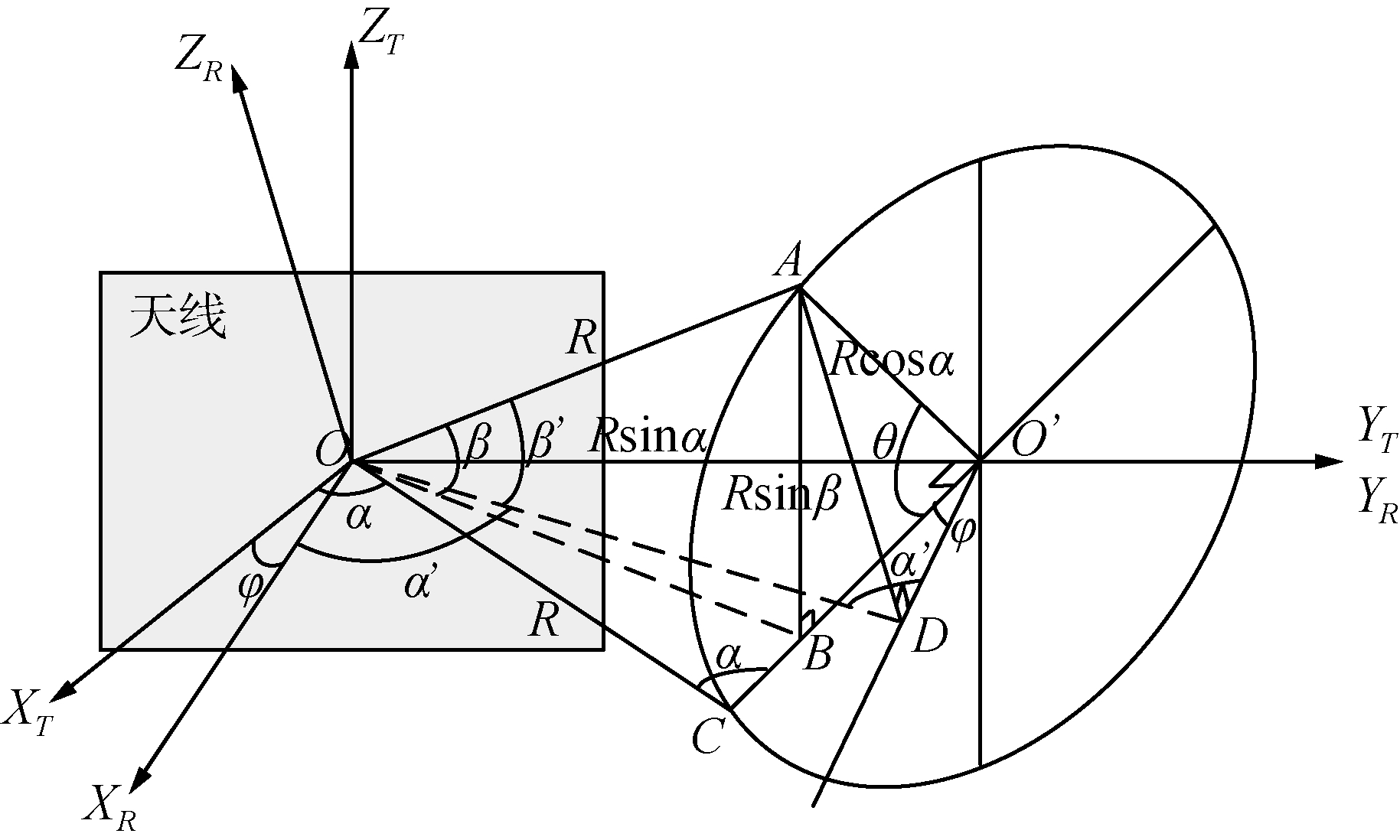Download: 图 4 倾斜角导致的测角误差几何关系 Fig. 4 Geometric relationship of the angle error due to radar tilt angle

2.3 天线测试方法导致的方位、俯仰测角误差研究

 $\left\{ \begin{array}{l} {\rm{sin}}\beta = {\rm{sin}}\theta {\rm{cos}}\alpha \\ \beta = {\rm{arcsin}}\left( {{\rm{sin}}\theta {\rm{cos}}\alpha } \right) \end{array} \right.$ (5)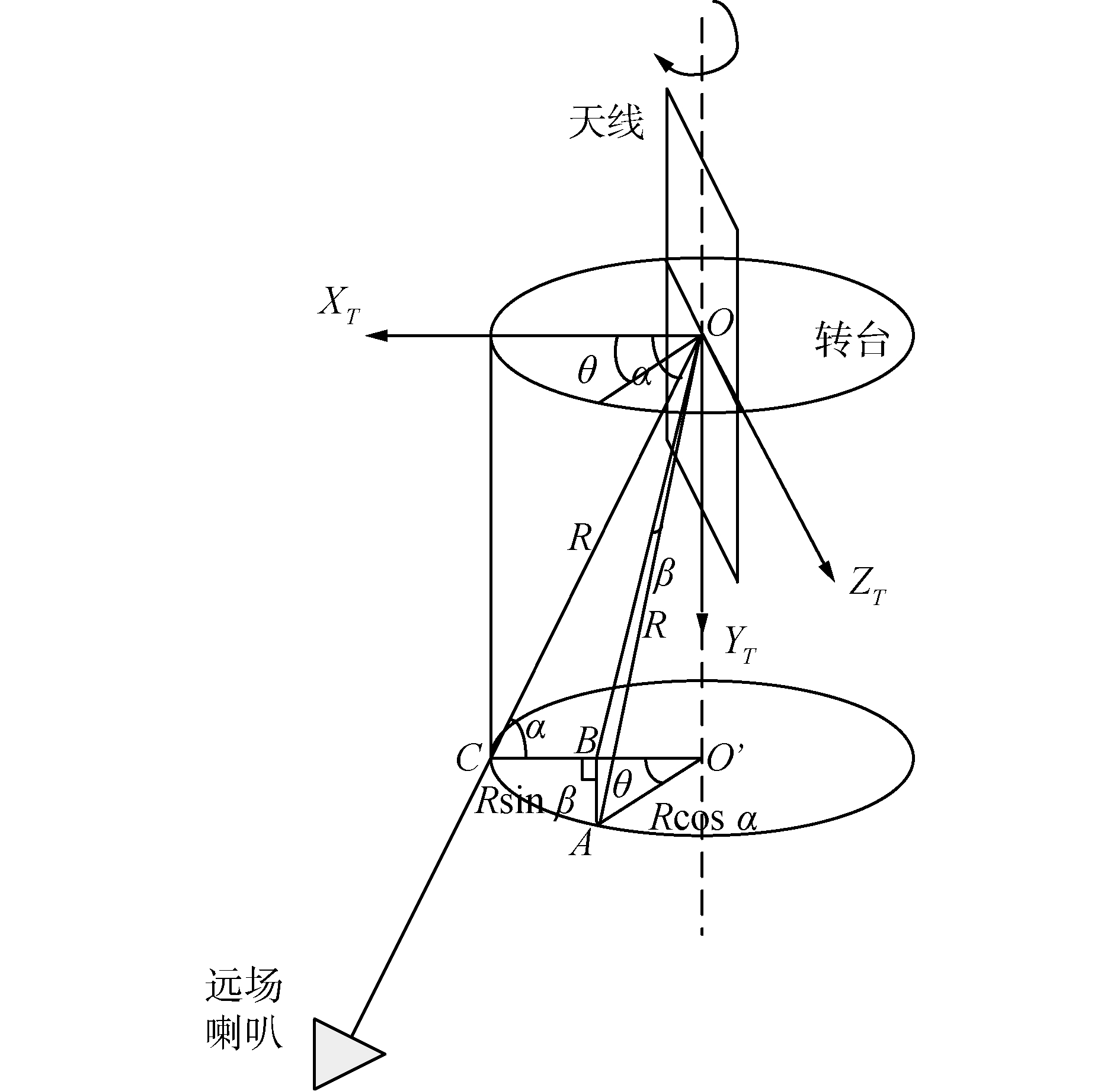Download: 图 5 雷达测试方法导致的测角误差几何关系 Fig. 5 Geometric relationship of the angle error due to radar test method

 $\alpha ^\prime = {\rm{arctan}}\left( {\frac{{{\rm{sin}}\alpha }}{{{\rm{cos}}\alpha {\rm{cos}}\left( {\beta + \varphi } \right)}}} \right)$ (6)
 $\beta ^\prime = {\rm{arcsin}}\left( {{\rm{cos}}\alpha {\rm{sin}}\left( {\beta + \varphi } \right)} \right)$ (7)

2.4 检测结果及分析Download: 图 6 修正前后参数变化 Fig. 6 Change of parameters before and after correction

3 结论

1) 方位测角误差和色散角、目标俯仰角有关，在色散角不为零的情况下，目标在天线阵面球坐标系下的方位测角误差会随着俯仰角的增大而变大，随着色散角与俯仰角的同时增大，方位测角误差将迅速增加。

2) 雷达球坐标系下测角误差和雷达倾斜角度有关，色散角、倾斜角度越大，方位、俯仰测角误差将随目标俯仰角度的增加而增大。

  李杰涛, 陈国际, 李伟, 等. DBF同时多波束测角方法研究及工程实现[J]. 火控雷达技术, 2013, 42(2): 19-22. LI Jietao, CHEN Guoji, LI Wei, et al. Study on DBF simultaneous multi-beam angle measuring method and its implementation in engineering[J]. Fire control radar technology, 2013, 42(2): 19-22. DOI:10.3969/j.issn.1008-8652.2013.02.006 (0)  朱伟, 陈伯孝, 周琦. 两维数字阵列雷达的数字单脉冲测角方法[J]. 系统工程与电子技术, 2011, 33(7): 1503-1509. ZHU Wei, CHEN Baixiao, ZHOU Qi. Angle measurement method with digital monopulse for2-dimensional digital array radar[J]. Systems engineering and electronics, 2011, 33(7): 1503-1509. DOI:10.3969/j.issn.1001-506X.2011.07.14 (0)  陈知明, 魏述刚. 相控阵雷达测角误差自适应计算方法[J]. 现代雷达, 2013, 35(7): 13-15. CHEN Zhiming, WEI Shugang. Self-adaptive calculation method of angel error curvemeasurement for phased array antenna[J]. Modern radar, 2013, 35(7): 13-15. DOI:10.3969/j.issn.1004-7859.2013.07.004 (0)  华瑶. 一种高效的高精度相控阵雷达工程测角方法[J]. 微波学报, 2016, 32(4): 88-91. HUA Yao. A high effective and high precision engineering calculation method of angel error curve measurement for phased array radar[J]. Journal of microwaves, 2016, 32(4): 88-91. (0)  张光义, 赵玉洁. 相控阵雷达技术[M]. 北京: 电子工业出版社, 2006: 12. (0)  SKOLNIK M I. Radar handbook[M]. 2nd ed. New York: McGraw-Hill, 1990. (0)  SKOLNIK M I.雷达手册[M].王军, 林强, 译.北京: 电子工业出版社, 2003: 7. SKOLNIK M I. Radar handbook[M]. WANG Jun, LIN Qiang, trans. Beijing: Publishing House of Electronics Industry, 2003: 7. (0)  郦能敬. 对空情报雷达的测量精度分析[J]. 雷达科学与技术, 2005, 3(1): 1-10. LI Nengjing. Analysis of measurement accuracy of air surveillance radar[J]. Radar science and technology, 2005, 3(1): 1-10. DOI:10.3969/j.issn.1672-2337.2005.01.001 (0)  温丹昊, 马敏, 刘志高. 相控阵雷达波束调度中的三维坐标转换方法[J]. 科技论坛, 2012(12): 25-26. (0)  张军, 刘衍, 赵迎超. 天线与转台之间的坐标关系[J]. 火控雷达技术, 2007, 36(1): 30-32. ZHANG Jun, LIU Yan, ZHAO Yingchao. Coordinate relation between antenna and turntable[J]. Fire control radar technology, 2007, 36(1): 30-32. DOI:10.3969/j.issn.1008-8652.2007.01.007 (0)  韩彦明, 陈希信. 自适应和差波束形成与单脉冲测角研究[J]. 现代雷达, 2010, 32(12): 44-47. HAN Yanming, CHEN Xixin. A study on adaptive sum and difference beamforming and monopulse angle estimation[J]. Modern radar, 2010, 32(12): 44-47. DOI:10.3969/j.issn.1004-7859.2010.12.010 (0)  NIELSEN R O. Accuracy of angle estimation with monopulse processing using two beams[J]. IEEE transactions on aerospace and electronic systems, 2001, 37(4): 1419-1423. DOI:10.1109/7.976976 (0)  SKOLNIK M I. Introduction to radar systems[M]. 3rded. New York: McGraw-Hill Education, 2002: 1-10. (0)  杨晓倩, 刘海波, 姜菡, 等. 基于数字阵列雷达的单脉冲测角技术研究[J]. 空军预警学院学报, 2013, 27(3): 184-187. YANG Xiaoqian, LIU Haibo, JIANG Han. Study of mono-pulse angle measurement based on digital array radar[J]. Journal of air force early warning academy, 2013, 27(3): 184-187. (0)  甘明. 基于相控阵天线单脉冲测角算法的测角精度研究[J]. 现代电子技术, 2013, 36(7): 89-93. GAN Ming. Research on precision of monopulse angle measurement based on phased array antenna[J]. Modern electronics technique, 2013, 36(7): 89-93. DOI:10.3969/j.issn.1004-373X.2013.07.028 (0)